# Glossary¶

This glossary gives an overview of how specific terms are used inside the EnMAP-Box.

All the terms that relate to GIS in general should be consistent with the terms given by the QGIS user manual and GUI. Because the EnMAP-Box integrates into the QGIS GUI, we try to not (as far as possible) contradict or redefine terminology.

All terms that relate to machine learning should be consistent with the definitions given by Scikit-Learn and the Scikit-Learn glossary, because we wildly crosslink into the Scikit-Learn docs!

Index

## GIS and Remote Sensing¶

attribute
A synonym for field.
attribute table
A tabulated data table associated with a vector layer. Table columns and rows are referred to as fields and geographic features respectively.
attribute value
Refers to a single cell value inside the attribute table of a vector layer.
band
A raster layer is composed of one or multiple bands.
categorized layer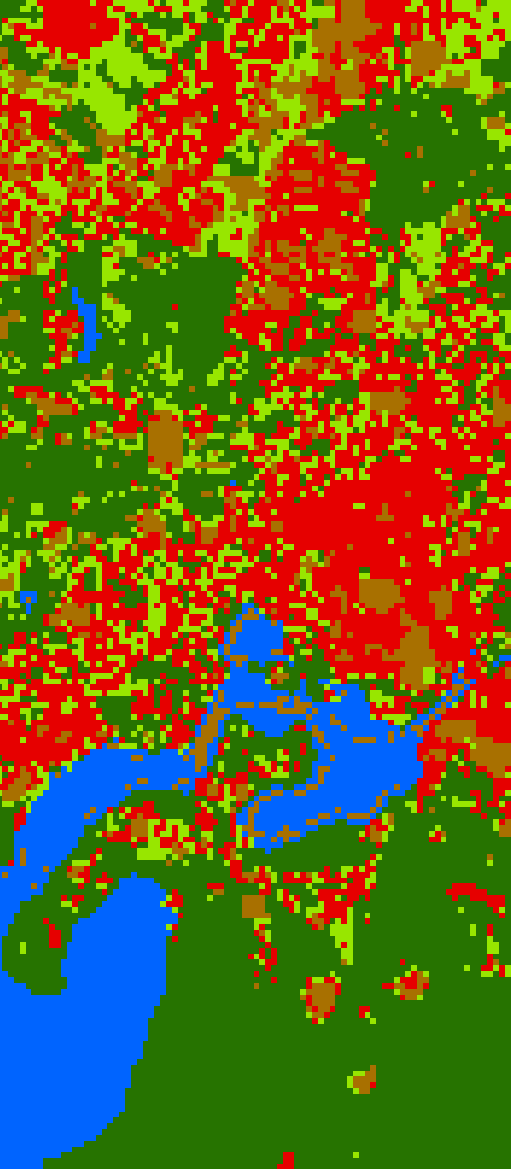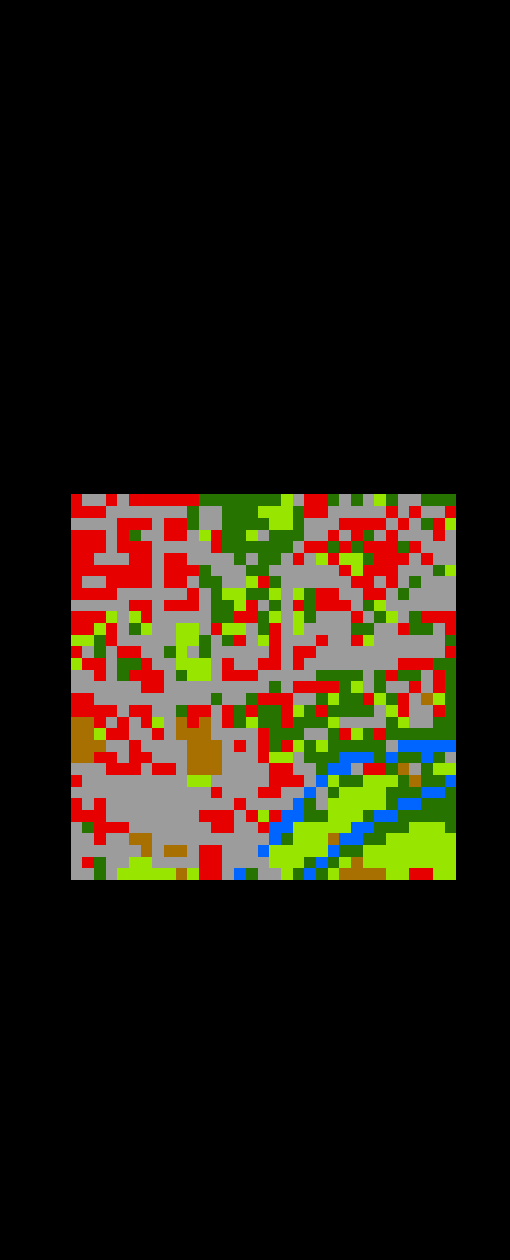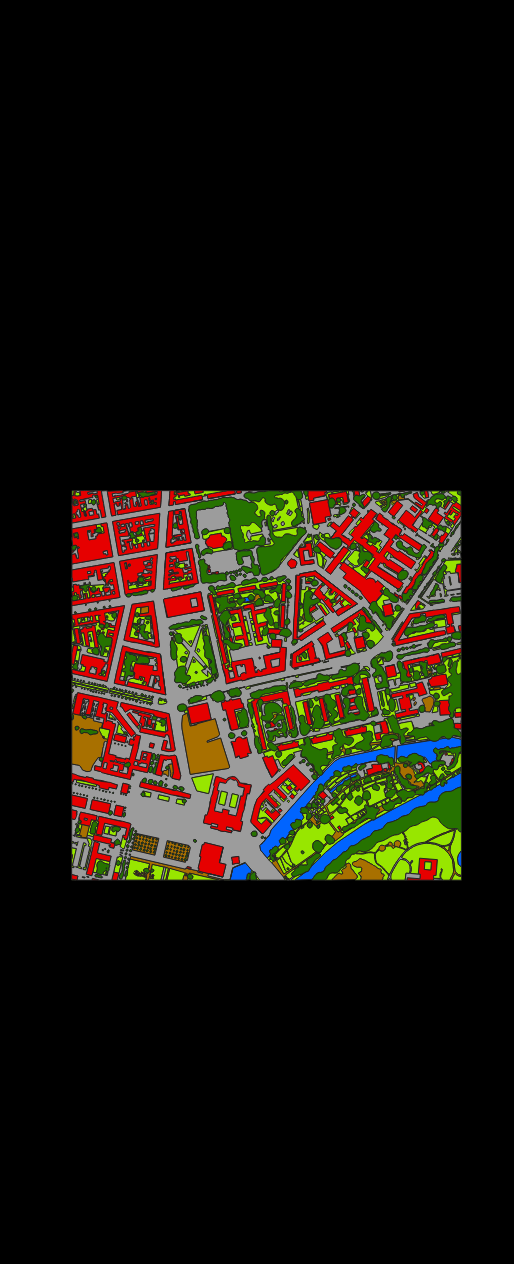categorized raster layer

A raster layer styled with a paletted/unique values renderer. The renderer defines the band with category values and a list of named and colored categories. Styles are usually stored as QML sidecar files. Category values don’t have to be strictly consecutive.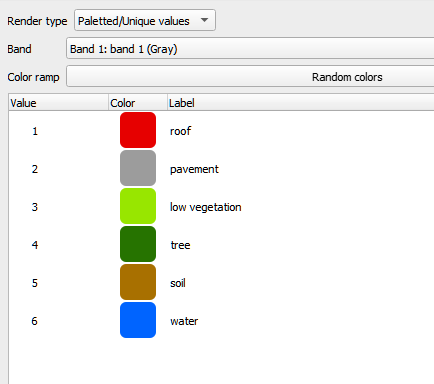categorized vector layer

A vector layer styled with a categorized symbol renderer. The renderer defines the field storing the category values (numbers or strings; expressions not yet supported) and a list of named and colored categories. Styles are usually stored as QML sidecar files. Note that in case of numerical category values, the values don’t have to be strictly consecutive.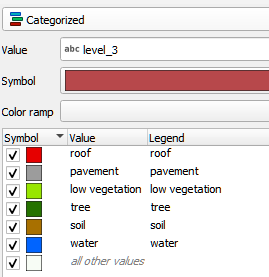categorized spectral library

A spectral library that is also a categorized vector layer.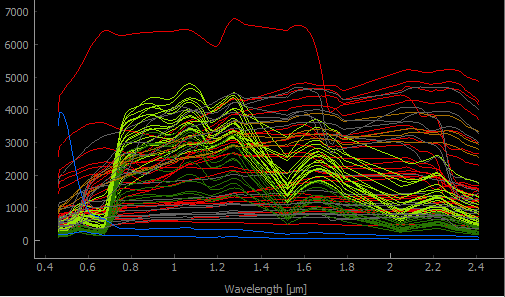category
categories
A category has a value, a name and a color.
class
Synonym for category.
classification layer

A categorized raster layer that is assumed to represent a mapping of a contiguous area.Note that there is currently no equivalent term for a contiguous vector polygon layer. We may introduce it in the future as needed. For now we expect users to rasterize such a vector layer into a raster layer.

class probability layer
A multi-band raster layer, where the bands represent class probabilities (values between 0 and 1) for a set of categories.
class fraction layer
A multi-band raster layer, where the bands represent class cover fractions (values between 0 and 1) for a set of categories.
color
An rgb-color, hex-color or int-color specified by a red, green and blue component. Learn more here: https://htmlcolorcodes.com/
continuous-valued raster layer

A raster layer, where each band represents a continuous-valued variable. Variable names are given by the raster band names.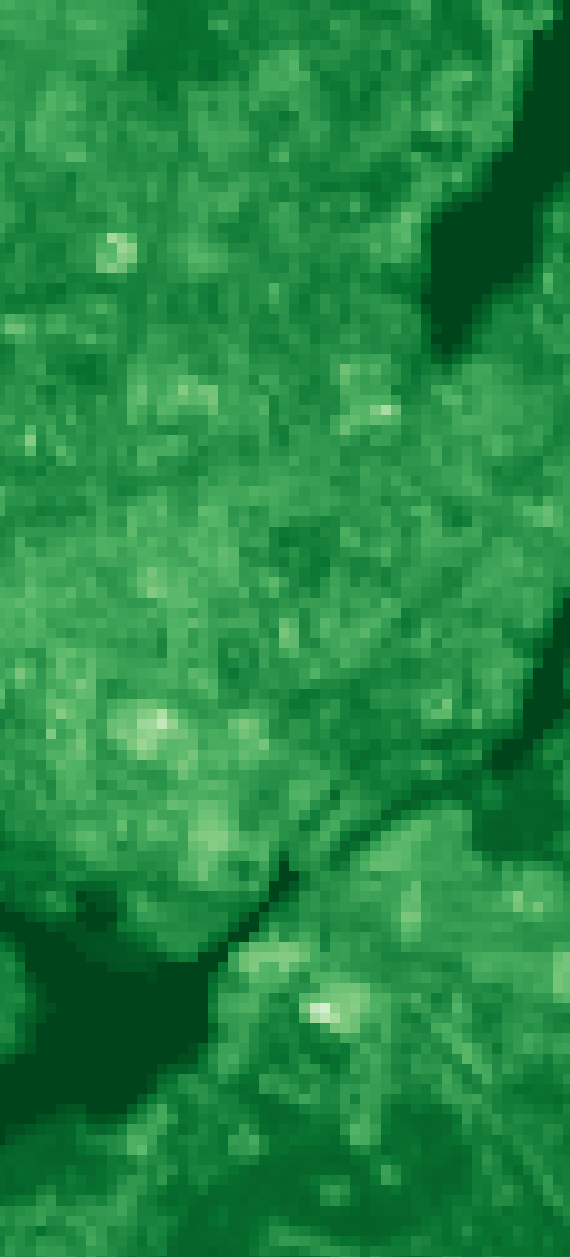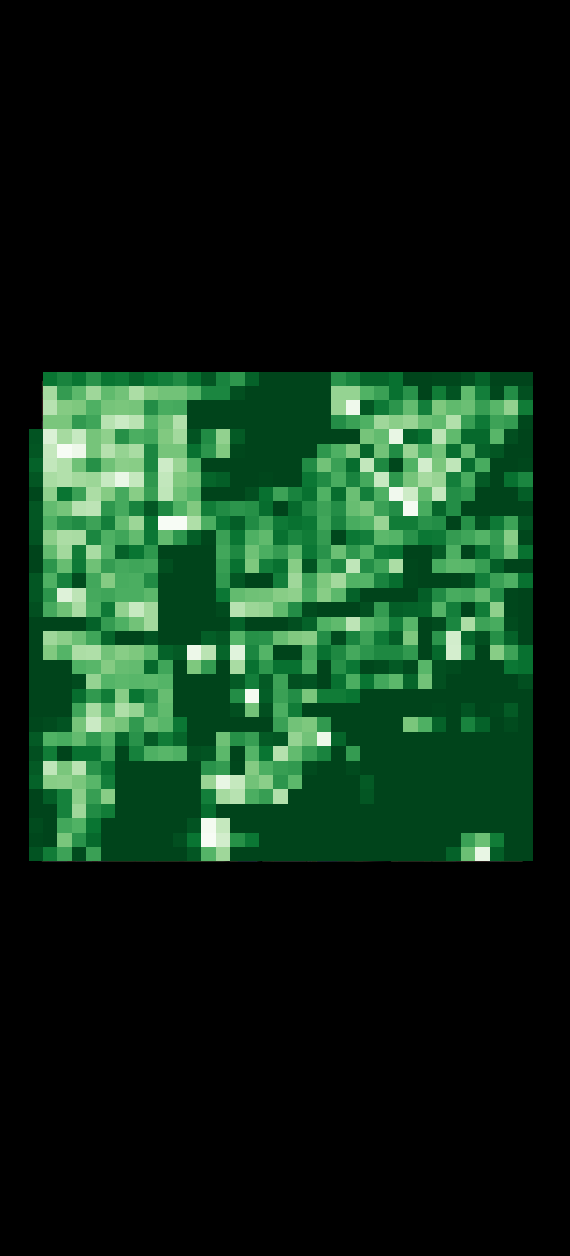continuous-valued vector layer

A vector layer with numeric fields representing continuous-valued variables. Variable names are given by field names.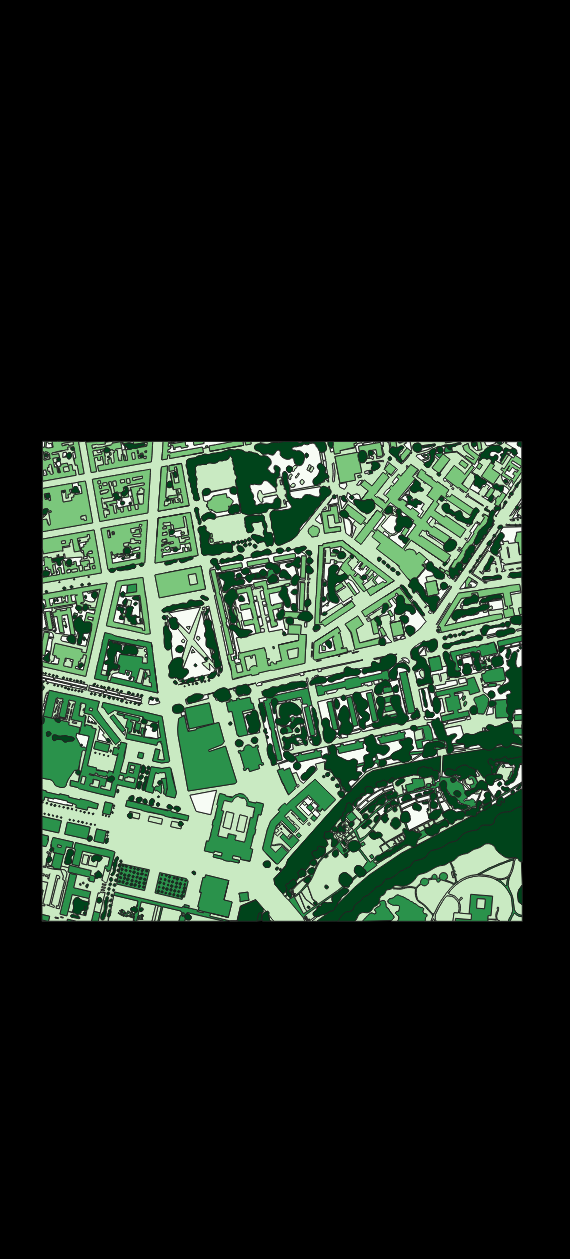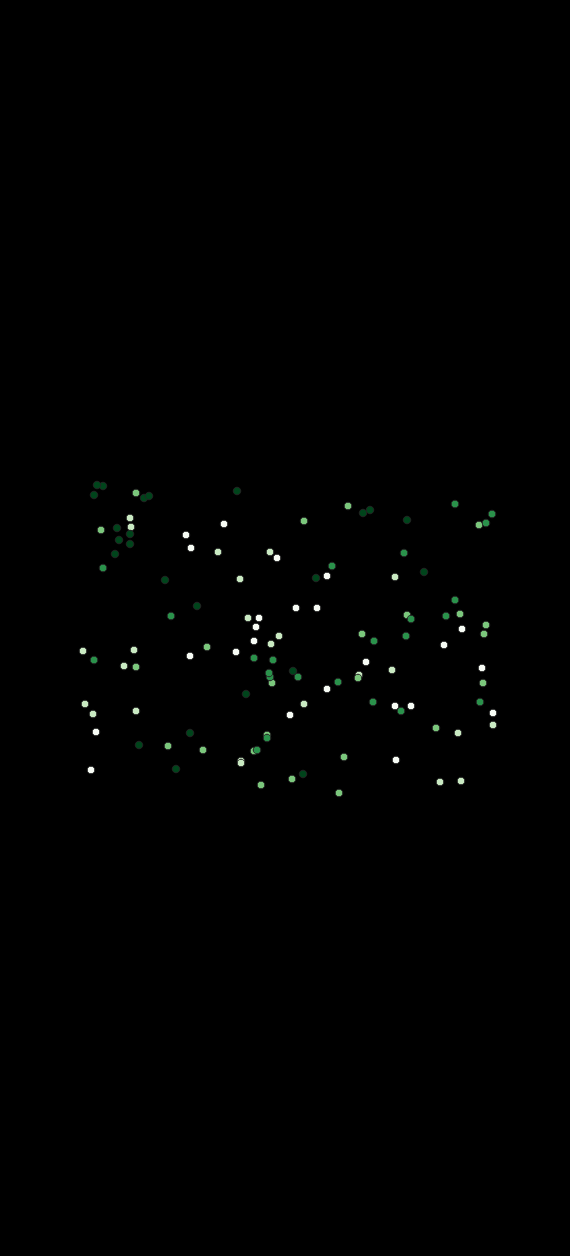continuous-valued layerfield
Refers to a single column inside the attribute table of a vector layer.
geographic feature

Refers to a single row inside the attribute table of a vector layer. In a vector layer, a geographic feature is a logical element defined by a point, polyline or polygon.

Note that in the context of GIS, the epithet “geographic” in “geographic feature” is usually skipped. In the context of EnMAP-Box, and machine learning in general, the term “feature” is used differently.

See feature for details.

grid
A raster layer defining the spatial extent, coordinate reference system and the pixel size.
hex-color
A color specified by a 6-digit hex-color string, where each color component is represented by a two digit hexadecimal number, e.g. red #FF0000, green #00FF00, blue #0000FF, black #000000, white #FFFFFF and grey #808080.
int-color
A color specified by a single integer between 0 and 256^3 - 1, which can also be represented as a hex-color.
labeled layer
A categorized layer or a continuous-valued layer.
layer
A vector layer or a raster layer.
layer style
The style of a layer can be defined in the Layer Styling panel and the Styling tab of the Layer Properties dialog. Some applications and algorithms take advantage of style information, e.g. for extracting category names and colors.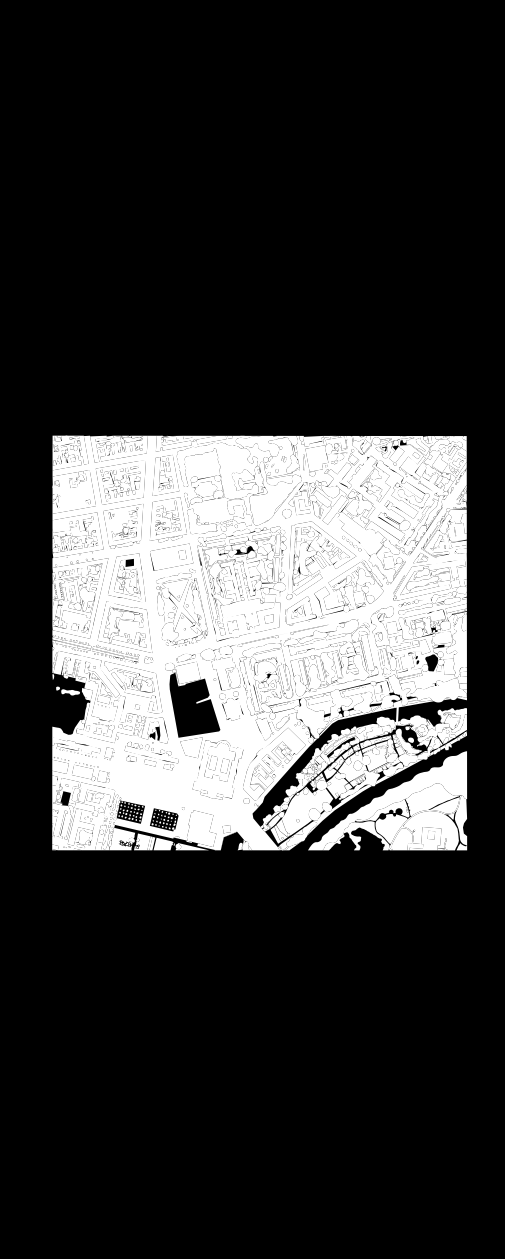A raster layer interpreted as a binary mask. All no data (zero, if missing), inf and nan pixel evaluate to false, all other to true. Note that only the first band used by the renderer is considered.A vector layer interpreted as a binary mask. Areas covered by a geometry evaluate to true, all other to false.pickle file

A binary file ending on .pkl that contains a pickled Python object, usually a dictionary or list container. Pickle file content can be browsed via the EnMAP-Box Data Sources panel: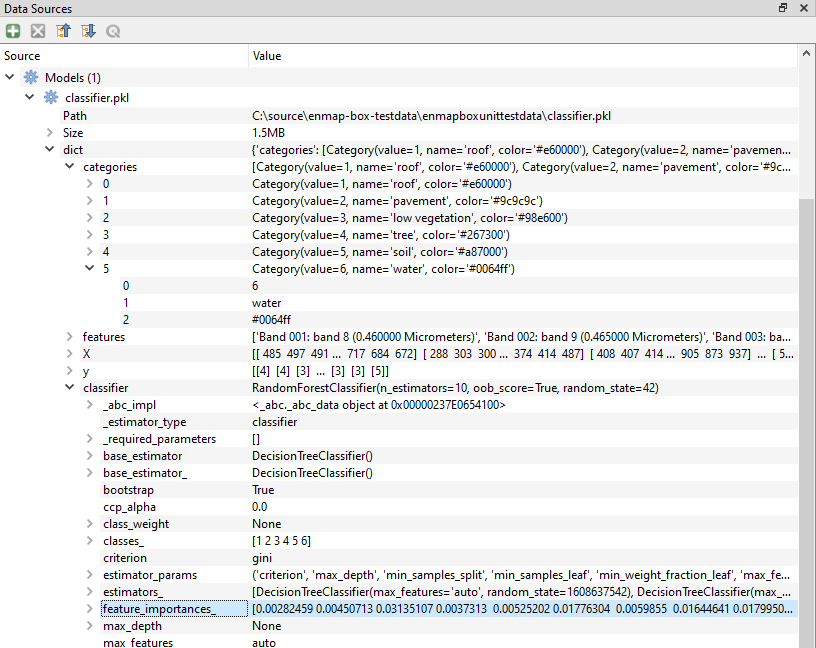pixel profile

List of band values for a single pixel in a raster layer.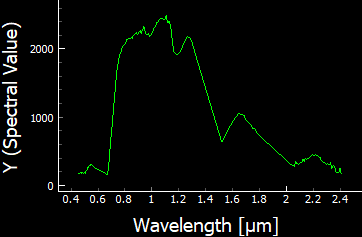point layer

A vector layer with point geometries.polygon layer

A vector layer with polygon geometries.ployline layer
A vector layer with line geometries.
raster layer

Any raster file that can be opened in QGIS as QgsRasterLayer. Elsewhere known as an image.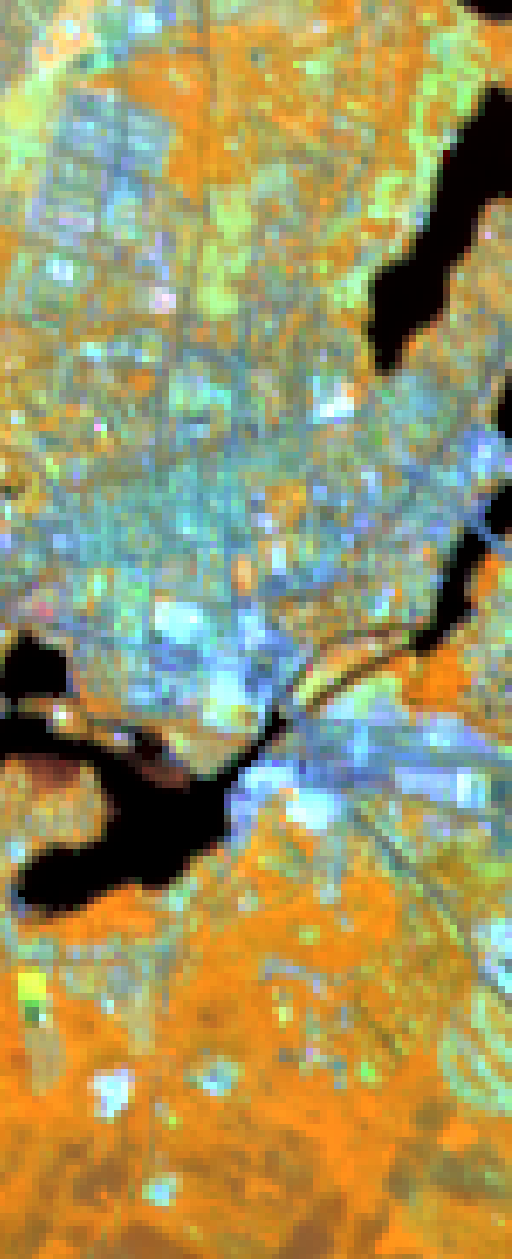regression layer

A continuous-valued raster layer that is assumed to represent a mapping of a contiguous area.rgb-color
A color specified by a triplet of byte values (values between 0 and 255) representing the red, green and blue color components, e.g. red (255, 0, 0), green (0, 255, 0), blue (0, 0, 255), black (0, 0, 0), white (255, 255, 255) and grey (128, 128, 128).
RGB image
A 3-band byte raster layer with values ranging from 0 to 255.
spectral band
A band inside a spectral raster layer. A spectral band represents a measurement for a region of the electromagnetic spectrum around a specific center wavelength. The region is typically described by a spectral response function.
spectral library

A vector layer with (at least) one special binary field containing pickled profile data and metadata. If a spectral library has exactly one such binary field, each geographic feature represents one spectral profile. In the case of n different binary fields, each geographic feature represents n profiles.

A spectral library is a collection of profiles with arbitrary profile-wise data and metadata, stored as pickled dictionaries inside (multiple) binary fields. Dictionary items are:

• x: list of x values (e.g. wavelength)
• y: list of y values (e.g. surface reflectance)
• xUnit: x value units (e.g. nanometers)
• yUnit: y value units (e.g. ???)
• bbl: the bad bands list

See enmapbox.externals.qps.speclib.core.SpectralLibrary for details.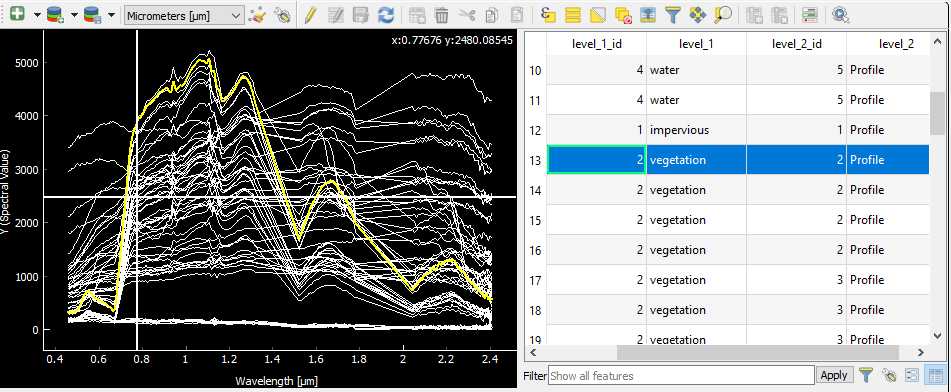spectral profile

A pixel profile in a spectral raster layer or a profile in a spectral library.spectral raster layer

A raster layer where the individual bands (i.e. spectral bands) represent measurements across the electromagnetic spectrum. The measurement vector of a single pixel is called a spectral profile)spectral response function
The spectral response describes the sensitivity of a sensor to optical radiation of different wavelengths.
spectral response function library

A spectral library, where each profile represents the spectral response function of a spectral band.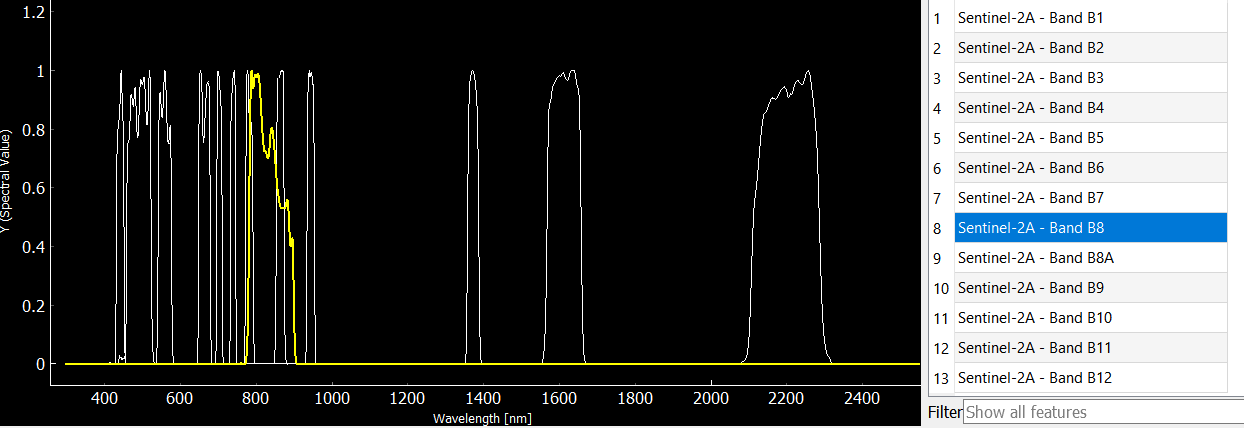stratification layer

A classification layer that is used to stratify an area into distinct subareas.stratum
strata
A category of a classifcation layer that is used as a stratification layer. Conceptually, a stratum can be seen as a binary mask with all pixels inside the stratum evaluating to True and all other pixels evaluating to False.
table

A vector layer with (potentially) missing geometry.

Note that in case of missing geometry, the vector layer icon looks like a table and layer styling is disabled.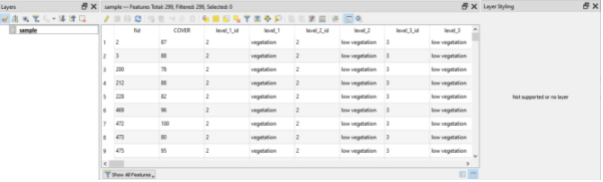vector feature
Synonym for geographic feature.
vector layer

Any vector file that can be opened in QGIS as QgsVectorLayer.band description
band name
Defined by GDAL data model. Accessible via gdal.Band.GetDescription().
The bad band multiplier value is indicating whether a band is usable (1) or not (0). Also see bad bands list for details.
bbl

List of bad band multiplier values of each band, typically 0 for bad bands and 1 for good bands.

Historically that information is stored in ENVI format and domain. Accessible via gdal.Dataset.GetMetadataItem(‘fwhm’, ‘ENVI’).

We store that information band-wise in the default domain. Accessible via gdal.Band.GetMetadataItem(‘bad band multiplier’).

center wavelength
A synonym for wavelength.
fwhm
full-width-at-half-maximum

List of full-width-half-maximum (FWHM) values of each band. Units should be the same as those used for wavelength and set in the wavelength units parameter.

Historically that information is stored in ENVI format and domain. Accessible via gdal.Dataset.GetMetadataItem(‘fwhm’, ‘ENVI’).

We store that information band-wise in the default domain. Accessible via gdal.Band.GetMetadataItem(‘fwhm’).

no data value
Defined by GDAL data model. Accessible via gdal.Band.GetNoDataValue().
wavelength

List of center wavelength values of each band. Units should be the same as those used for the fwhm and set in the wavelength units parameter.

Historically that information is stored in ENVI format and domain. Accessible via gdal.Dataset.GetMetadataItem(‘wavelength’, ‘ENVI’).

We store that information band-wise in the default domain. Accessible via gdal.Band.GetMetadataItem(‘wavelength’).

wavelength units

Text string indicating one of the following wavelength units: Micrometers, um, Nanometers, nm, Index, Unknown

Historically that information is stored in ENVI format and domain. Accessible via gdal.Dataset.GetMetadataItem(‘wavelength units’, ‘ENVI’).

We store that information band-wise in the default domain. Accessible via gdal.Band.GetMetadataItem(‘wavelength units’).

## Machine Learning¶

EnMAP-Box provides nearly all of it’s machine learning related functionality by using Scikit-Learn in the background. So we decided to also adopt related terminology and concepts as far as possible, while still retaining the connection to GIS and remote sensing in the broader context of being a QGIS plugin. Most of the following definitions are directly taken from the Scikit-Learn glossary as is, and only expanded if necessary.

classification
The process of identifying which category an object belongs to.
classifier
A supervised estimator with a finite set of discrete possible output values.
clusterer
An unsupervised estimator with a finite set of discrete output values.
clustering
The process of automatic grouping of similar objects into sets.
cross-validation

The training dataset is split into k smaller sets and the following procedure is followed for each of the k “folds”:

• a model is trained using k-1 of the folds as training dataset
• the resulting model is used to predict the targets of the remaining part of the dataset

The performance can now be calculated from the predictions for the whole training dataset.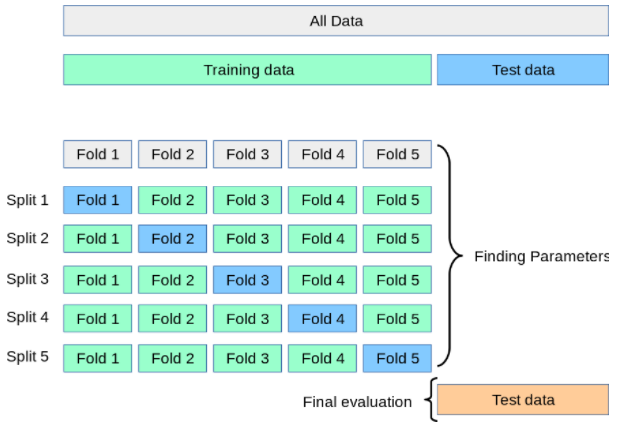This approach can be computationally expensive, but does not waste too much data (as is the case when fixing an arbitrary validation set), which is a major advantage in problems where the number of samples is very small.

dataset

A dataset is a complete representation of a learning problem, combining feature data X and target data y. Datasets are often splitted into sub-datasets. One common splitting technique is the train-test split, where a part of the dataset is held out as a so-called training dataset used for fitting the estimator and another part is held out as a test dataset used for a final evaluation.

When evaluating different settings (i.e. hyperparameters) for an estimator, yet another part of the dataset can be held out as a so-called validation dataset. Training proceeds on the training dataset, best parameters are found by evaluating against the validation dataset, and final evaluation can be done on the test dataset. Holding out a validation datase can be avoided by using cross-validation for hyperparameter tuning.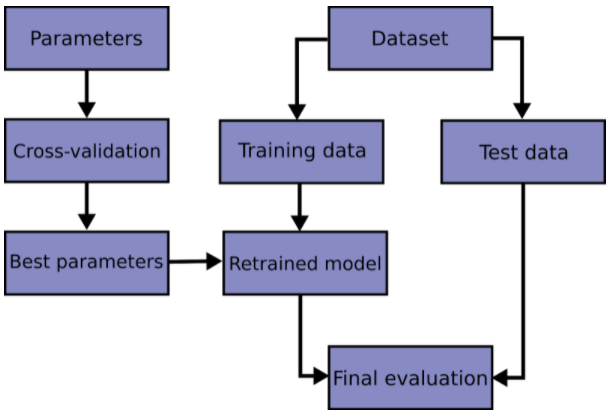estimator
An object which manages the estimation of a model. The model is estimated as a deterministic function.
evaluation metric

Evaluation metrics give a measure of how well a model (e.g. a classifier or regressor) performs.

feature
feature vector

In QGIS and other GIS, the term feature is well defined as a logical element defined by a point, polyline or polygon inside a vector layer. In the context of the EnMAP-Box, we refere to it as geographic feature.

In machine learning, a feature is a component in a so-called feature vector, which is a list of numeric quantities representing a sample in a dataset. A set of samples with feature data X and associated target data y or Y form a dataset.

Elsewhere features are known as attributes, predictors, regressors, or independent variables. Estimators assume that features are numeric, finite and not missing. n_features indicates the number of features in a dataset.

n_features
The number of features in a dataset.
n_outputs
The number of outputs in a dataset.
n_samples
The number of samples in a dataset.
n_targets
Synonym for n_outputs.
output

Individual scalar/categorical variables per sample in the target.

Also called responses, tasks or targets.

regression
The process of predicting a continuous-valued attribute associated with an object.
regressor
A supervised estimator with continuous output values.
sample

We usually use this term as a noun to indicate a single feature vector.

Elsewhere a sample is called an instance, data point, or observation. n_samples indicates the number of samples in a dataset, being the number of rows in a data array X.

target

The dependent variable in supervised learning, passed as y to an estimator’s fit method.

Also known as dependent variable, outcome variable, response variable, ground truth or label.

test dataset
The dataset used for final evaluation.
training dataset
The dataset used for training.
transformer
An estimator that transforms the input, usually only feature data X, into some transformed space (conventionally notated as Xt).
validation dataset
The dataset used for finding best parameters (i.e. hyperparameter tuning).
X
Denotes data that is observed at training and prediction time, used as independent variables in learning. The notation is uppercase to denote that it is ordinarily a matrix.
y
Y
Denotes data that may be observed at training time as the dependent variable in learning, but which is unavailable at prediction time, and is usually the target of prediction. The notation may be uppercase to denote that it is a matrix, representing multi-output targets, for instance; but usually we use y and sometimes do so even when multiple outputs are assumed.